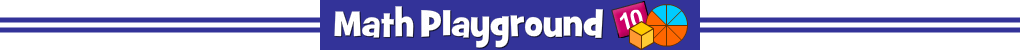Thinking Blocks Fractions - Learning Connections
Essential Skills
Problem Solving - model and solve word problems

Common Core Connection for Grades 3, 4 and 5
Understand a fraction a/b with a > 1 as a sum of fractions 1/b.
Join and separate parts referring to the same whole.
Solve word problems involving addition and subtraction of fractions
referring to the same whole and having like denominators.
Solve word problems involving multiplication of a fraction by a whole number.
More Math Games to Play